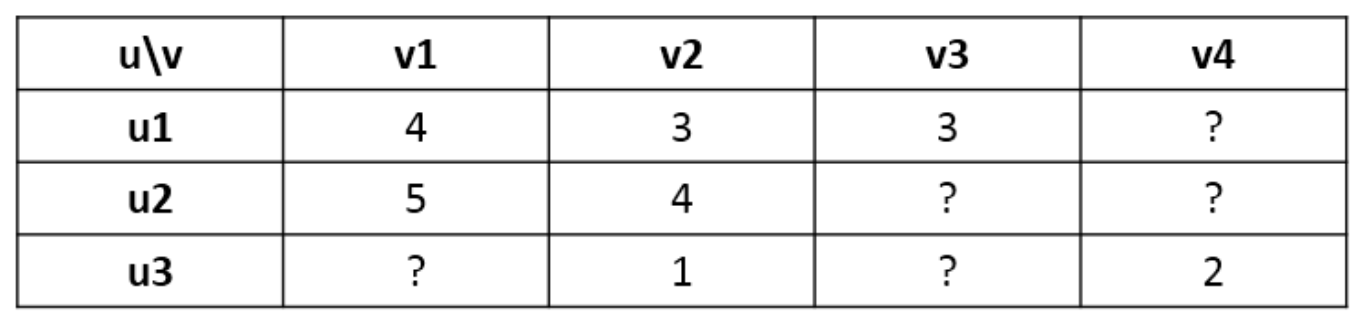# 基于协同的ALS算法原理介绍与实现

Github：https://github.com/thinkgamer

ALS也是一种协同算法，其全称是交替最小二乘法（Alternating Least Squares），由于简单高效，已被广泛应用在推荐场景中，目前已经被集成到Spark MLlib和ML库中，在下一篇文章会对其使用方式进行详细介绍，本篇文章主要介绍ALS的底层算法原理。

### 最小二乘法（Least Squares）

LS也被用于拟合曲线，比如所熟悉的线性模型。下面以简单的线性一元线性回归模型说明最小二乘法。

$y = w_0 + w_1 x$

$L_n = (y_n - f(x;w_0,w_1))^2$

$L = \frac{1}{N} \sum_{n=1}^{N} (y_n - f(x;w_0,w_1))^2$
L越小表示参数w越精确，而这里最关键的就是寻找到最合适的w0和w1，则此时的数学表达式为：
$\underset{w_0,w_1}{arg \ min} \frac{1}{N} \sum_{n=1}^{N} (y_n - f(x;w_0,w_1))^2$

$L = \frac{1}{N} \sum_{n=1}^{N} (y_n - w_0 - w_1 x_n)^2 \\ \frac{1}{N} \sum_{n=1}^{N} (w_1 ^2x_n^2 + 2w_1x_n(w_0 - y_n) + w_0^2 - 2w_0y_n + y_n^2)$
L函数取得最小值时，w0和w1的一阶偏导数一定是0（因为误差平方和是一个大于等于0的数，是没有最大值的，所以取得最小值时，一阶偏导数一定为0）。因为对L函数分别求偏导，使其等于0，并对w0和w1求解，即可。

### 交替最小二乘法（Alternating Least Squares）

ALS算法本质上是基于物品的协同，近年来，基于模型的推荐算法ALS(交替最小二乘)在Netflix成功应用并取得显著效果提升，ALS使用机器学习算法建立用户和物品间的相互作用模型，进而去预测新项。

#### 基本原理#### 模型抽象

$R \approx UV^T$

• R为打分矩阵（m*n，m表示用户个数，n表示物品个数）
• U表示用户对隐含特征的偏好矩阵（m*k）
• V表示物品对隐含特征的归属矩阵（n*K）

#### 代价函数

$J(U,V) = \sum_{i}^{m} \sum_{j}^{n}[(r_{ij} - u_iv_j^T) ^2 + \lambda ( ||u_i||^2 + ||v_j||^2 ) ]$

#### 隐式反馈代价函数

$p_{ij} = \left\{\begin{matrix} 1 & r_{ij} >0 \\ 0 & r_{ij} =0 \end{matrix}\right.$

$c_{ij} = 1 + \alpha r_{ij}$
α 为置信度系数，那么代价函数变为如下形式：
$J(U,V) = \sum_{i}^{m} \sum_{j}^{n}[c_{ij}(p_{ij} - u_iv_j^T) ^2 + \lambda ( ||u_i||^2 + ||v_j||^2 ) ]$

#### 算法求解

$U^0 \rightarrow V^1 \rightarrow U^1 \rightarrow V^2 \rightarrow U^2 ....$

$U ^T = \left( V^T V + \lambda I \right)^{-1} V^T R^T$

$V ^T = \left( U^T U + \lambda I \right)^{-1} U^T R$

$U ^T = \left( V^T C_v V + \lambda I \right)^{-1} V^T C_v R^T$

$V ^T = \left( U^T C_u U + \lambda I \right)^{-1} U^T C_u R$

### 面试点

1. 最小二乘法英文名字是什么？解释及其对应的数学原理
Least Squares


1. ALS全称是什么？为什么叫交替最小二乘法？
Alternaing Least Squares


1. 隐式反馈和显氏反馈的区别？两种形式下ALS的代价函数
《推荐系统开发实战》中有对其的介绍
两种形式下的代价函数参考上文

1. 代价函数中的正则项及其含义？
参考上文

1. 过拟合和欠拟合的含义？在ALS中什么情况会出现过拟合和欠拟合？对应的解决办法？
欠拟合定义：拟合的函数与训练集误差较大，


1. Spark实现ALS可调节的参数有哪些？分别表示什么含义？
maxIters // 最大迭代次数，默认10
rank // 隐向量的长度，默认是10，一般远小于m，n
numBlocks // 数据分区的个数，默认是10
regParam // ALS中的正则化参数，默认是1.0
alpha // ALS隐氏反馈变量的参数，置信度系数，默认是1.0
userCol // 用户列名
itemCol // item列名
rateCol // 评分列名
implicitPrefs // 显氏反馈 还是 隐氏反馈，默认false，意味显氏反馈【搜索与推荐Wiki】专注于搜索和推荐系统，尝试使用算法去更好的服务于用户，包括但不局限于机器学习，深度学习，强化学习，自然语言理解，知识图谱，还不定时分享技术，资料，思考等文章！